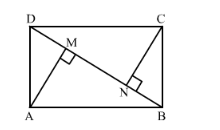# ABCD is a rectangle. Points M and N are on BD such that AM ⊥ BD and CN ⊥ BD.

Question:

$A B C D$ is a rectangle. Points $M$ and $N$ are on $B D$ such that $A M \perp B D$ and $C N \perp B D$. Prove that $B M^{2}+B N^{2}=D M^{2}+D N^{2}$.

Solution:

Given: A rectangle ABCD where AM  BD and CN  BD.

To prove: $\mathrm{BM}^{2}+\mathrm{BN}^{2}=\mathrm{DM}^{2}+\mathrm{DN}^{2}$

Proof:Apply Pythagoras Theorem in $\triangle \mathrm{AMB}$ and $\triangle \mathrm{CND}$,

$\mathrm{AB}^{2}=\mathrm{AM}^{2}+\mathrm{MB}^{2}$

$\mathrm{CD}^{2}=\mathrm{CN}^{2}+\mathrm{ND}^{2}$

Since $A B=C D, A M^{2}+M B^{2}=C N^{2}+N D^{2}$

$\Rightarrow \mathrm{AM}^{2}-\mathrm{CN}^{2}=\mathrm{ND}^{2}-\mathrm{MB}^{2} \ldots$ (i)

Again apply Pythagoras Theorem in $\triangle A M D$ and $\triangle C N B$,

$\mathrm{AD}^{2}=\mathrm{AM}^{2}+\mathrm{MD}^{2}$

$\mathrm{CB}^{2}=\mathrm{CN}^{2}+\mathrm{NB}^{2}$

Since $A D=B C, A M^{2}+M D^{2}=C N^{2}+N B^{2}$

$\Rightarrow \mathrm{AM}^{2}-\mathrm{CN}^{2}=\mathrm{NB}^{2}-\mathrm{MD}^{2} \ldots$ (ii)

Equating (i) and (ii),

$\mathrm{ND}^{2}-\mathrm{MB}^{2}=\mathrm{NB}^{2}-\mathrm{MD}^{2}$

I.e., $B M^{2}+B N^{2}=D M^{2}+D N^{2}$

This proves the given relation.